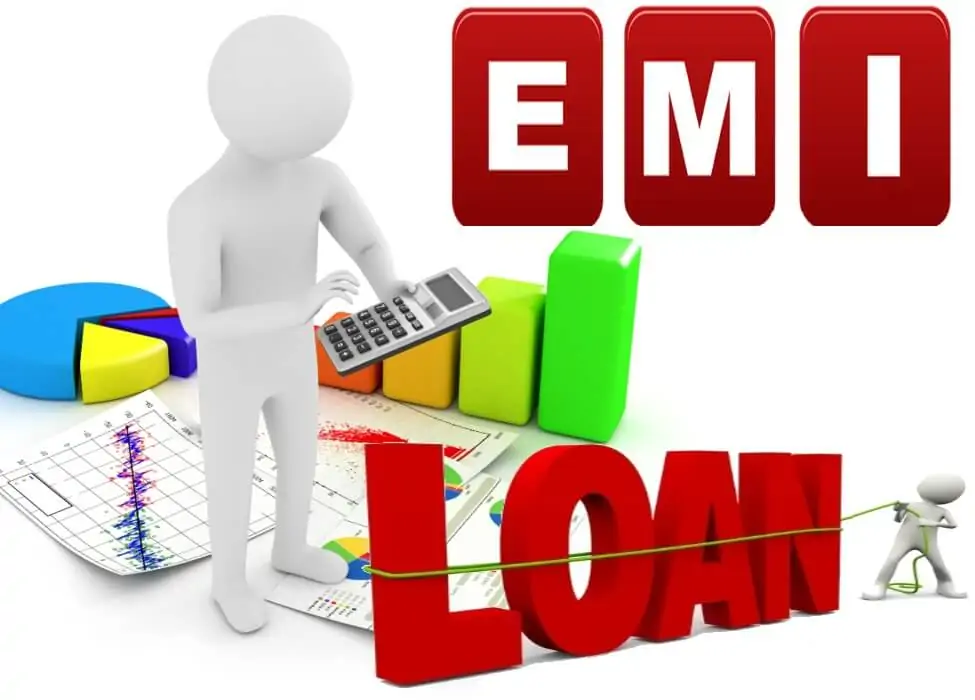# EMI Loan Calculator | Amortization Schedule Calculator

This is all in one EMI Loan Calculator with prepayment, chart, amortization table and lot of additional feature specially for you. This EMI Loan Amortization Calculator can be used as Home Loan Calculator, Home Renovation Loan EMI Calculator, Personal EMI Calculator, Amortization Schedule Calculator, Car EMI Calculator and Two-Wheeler Loan EMI Calculator. Apart from these simple calculator, it can also be used as Payday Loan Calculator, Credit Card EMI Calculator, Small Business Loan Calculator, Agriculture Loan Calculator, Gold Loan EMI Calculator, Education Loan Calculator, Consumer Durable Loan Calculator and many more types of calculators.

Note: (1) There are lot of differences in excel sheet and google spreadsheet. Due to which we have created separate version for different spreadsheets. (2) You need to click on “Enable Editing” when you see “Protected View” default error message in excel spreadsheet.

## What is Loan?

Loan means providing money by banks, associations, institutions, person to other person, organization and so forth. The beneficiary (called as borrower) is obligated to pay interested on loan amount until principle is repaid to then lender. In simple words, a loan is defined as asset, money or material products given to another entity in return for future repay of loan given along with interest and any other processing charges.

Borrowed money is known as principle and interest is the sum charged for getting the loan in financial terms. There are broadly two types of Loans and they are secured loan and unsecured loan. Secured loan involves lesser risk to lender. As borrower need to pledge its assets as collateral to receive a loan from bank or any other financial institution. These assets can be either vehicle, property, gold and many more similar things. When borrower fails to repay the loan, lender possess rights to sell the asset to recover its loan amount along with principle. Unsecured loan means lender do not get any rights. In the event when borrower fails to repay loan, the moneylender doesn’t reserve any option to take anything legal action against borrower. It is advisable to compare mortgage from loan comparison calculator, if you have not applied for any loan.

## What is EMI?

An EMI is defined as a fixed installment payment decided between borrower and lender at a predetermined schedule date for each month until the loan amount is repaid. Equated Monthly Installment is a full form for EMI. EMI includes principle and interest payments over a predefined number of years.

It is calculated in such a way that interest contribution is comparatively greater than principle during initial months. Gradually interest component keeps on reducing with every installment.

### EMI Calculation

EMI (Equated Monthly Installment) is calculated based on a Simple Formula as mentioned below:

EMI (Equated Monthly Installment) = (Principal X Interest Rate/12) X [(1+ Interest Rate/12) ^ Loan Tenure] / [(1+ Interest Rate/12) ^ Loan Tenure-1].

Let us take an EMI Calculation example to understand better.

Assume that you borrow 5,000,000 from the bank at 9% annual interest rate for a period of 20 years (Here Loan Tenure is 20/12 = 240 months). Let us now calculate Equated Monthly Installment based on this information.

EMI = (5,000,000 X 9/12) X [(1+ 9/12) ^ 240] / [(1+ 9/12) ^ 240-1]

EMI = (5,000,000 X 0.75) X [(1+ 0.75) ^ 240] / [(1+ 0.75) ^ 240-1]

EMI = 44,986

Here, total repayment amount will be 44,986 X 240 = 10,796,711 which includes 10,796,711 – 5,000,000 = 5,796,711 as interest payable toward the loan amount.

## How to Use Loan Amortization Calculator?

It is really simple to use it. You just need to enter few loan details like: Loan Amount, Annual Interest Rate, Loan Period, Payment Method, Start Date of Loan. Enter basic loan details to view the result and amortization schedule calculator. You can see the updated result in amortization table, chart and loan summary section. They will show the data based on your input values.

EMI Loan Calculator can be use to calculate any prepayment made during the loan tenure. Tool is much beneficial to personal financial advisors and finance related businesses. You are not allow to re-brand, sell or sell with customization without our written permission. Tool is 100% free to be use for personal and internal purpose.

## Who can Use EMI Calculator?

Here we have provided Loan Calculator with interactive amortization table and colourful charts. You can use this all in one EMI calculator as below calculators.

• Personal Loan Calculator
• Amortization Schedule Calculator
• Home Loan EMI Calculator / House EMI Calculator
• Car Loan Calculator / Vehicle Loan EMI Calculator
• Two-Wheeler Loan Calculator / Bike EMI Calculator
• Home Renovation Loan EMI Calculator
• Payday Loan Calculator
• Credit Card Loan Calculator